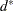The CLUSTER Procedure
 Displayed Output

If you specify the SIMPLE option and the data are coordinates, PROC CLUSTER produces simple descriptive statistics for each variable:

• the Mean

• the standard deviation, Std Dev

• the Skewness

• the Kurtosis

• a coefficient of Bimodality

If the data are coordinates and you do not specify the NOEIGEN option, PROC CLUSTER displays the following:

• the Eigenvalues of the Correlation or Covariance Matrix

• the Difference between successive eigenvalues

• the Proportion of variance explained by each eigenvalue

• the Cumulative proportion of variance explained

If the data are coordinates, PROC CLUSTER displays the Root Mean Squared Total-Sample Standard Deviation of the variables

If the distances are normalized, PROC CLUSTER displays one of the following, depending on whether squared or unsquared distances are used:

• the Root Mean Squared Distance Between Observations

• the Mean Distance Between Observations

For the generations in the clustering process specified by the PRINT= option, PROC CLUSTER displays the following:

• the Number of Clusters or NCL

• the names of the Clusters Joined. The observations are identified by the formatted value of the ID variable, if any; otherwise, the observations are identified by OB, whereis the observation number. The CLUSTER procedure displays the entire value of the ID variable in the cluster history instead of truncating at 16 characters. Long ID values might be split onto several lines. Clusters of two or more observations are identified as CL, whereis the number of clusters existing after the cluster in question is formed.

• the number of observations in the new cluster, Frequency of New Cluster or FREQ

If you specify the RMSSTD option and the data are coordinates, or if you specify METHOD=AVERAGE, METHOD=CENTROID, or METHOD=WARD, then PROC CLUSTER displays the root mean squared standard deviation of the new cluster, RMS Std of New Cluster or RMS Std.

PROC CLUSTER displays the following items if you specify METHOD=WARD. It also displays them if you specify the RSQUARE option and either the data are coordinates or you specify METHOD=AVERAGE or METHOD=CENTROID.

• the decrease in the proportion of variance accounted for resulting from joining the two clusters, Semipartial R-Squared or SPRSQ. This equals the between-cluster sum of squares divided by the corrected total sum of squares.

• the squared multiple correlation, R-Squared or RSQ. R square is the proportion of variance accounted for by the clusters.

If you specify the CCC option and the data are coordinates, PROC CLUSTER displays the following:

• Approximate Expected R-Squared or ERSQ, the approximate expected value of R square under the uniform null hypothesis

• the Cubic Clustering Criterion or CCC. The cubic clustering criterion and approximate expected R square are given missing values when the number of clusters is greater than one-fifth the number of observations.

If you specify the PSEUDO option and the data are coordinates, or if you specify METHOD=AVERAGE, METHOD=CENTROID, or METHOD=WARD, then PROC CLUSTER displays the following:

• Pseudoor PSF, the pseudostatistic measuring the separation among all the clusters at the current level

• Pseudoor PST2, the pseudostatistic measuring the separation between the two clusters most recently joined

If you specify the NOSQUARE option and METHOD=AVERAGE, PROC CLUSTER displays the (Normalized) Average Distance or (Norm) Aver Dist, the average distance between pairs of objects in the two clusters joined with one object from each cluster.

If you do not specify the NOSQUARE option and METHOD=AVERAGE, PROC CLUSTER displays the (Normalized) RMS Distance or (Norm) RMS Dist, the root mean squared distance between pairs of objects in the two clusters joined with one object from each cluster.

If METHOD=CENTROID, PROC CLUSTER displays the (Normalized) Centroid Distance or (Norm) Cent Dist, the distance between the two cluster centroids.

If METHOD=COMPLETE, PROC CLUSTER displays the (Normalized) Maximum Distance or (Norm) Max Dist, the maximum distance between the two clusters.

If METHOD=DENSITY or METHOD=TWOSTAGE, PROC CLUSTER displays the following:

• Normalized Fusion Density or Normalized Fusion Dens, the value ofas defined in the section Clustering Methods

• the Normalized Maximum Density in Each Cluster joined, including the Lesser or Min, and the Greater or Max, of the two maximum density values

If METHOD=EML, PROC CLUSTER displays the following:

• Log Likelihood Ratio or LNLR

• Log Likelihood or LNLIKE

If METHOD=FLEXIBLE, PROC CLUSTER displays the (Normalized) Flexible Distance or (Norm) Flex Dist, the distance between the two clusters based on the Lance-Williams flexible formula.

If METHOD=MEDIAN, PROC CLUSTER displays the (Normalized) Median Distance or (Norm) Med Dist, the distance between the two clusters based on the median method.

If METHOD=MCQUITTY, PROC CLUSTER displays the (Normalized) McQuitty’s Similarity or (Norm) MCQ, the distance between the two clusters based on McQuitty’s similarity method.

If METHOD=SINGLE, PROC CLUSTER displays the (Normalized) Minimum Distance or (Norm) Min Dist, the minimum distance between the two clusters.

If you specify the NONORM option and METHOD=WARD, PROC CLUSTER displays the Between-Cluster Sum of Squares or BSS, the ANOVA sum of squares between the two clusters joined.

If you specify neither the NOTIE option nor METHOD=TWOSTAGE or METHOD=DENSITY, PROC CLUSTER displays Tie, where a T in the column indicates a tie for minimum distance and a blank indicates the absence of a tie.

After the cluster history, if METHOD=TWOSTAGE or METHOD=DENSITY, PROC CLUSTER displays the number of modal clusters.Previous Page | Next Page | Top of Page